I realized that I owe you something. In a prior post, I invoked some Bayesian ideas to contrast with boostrapping analysis of high-variance data. (More precisely, it was high log-variance data for which there was a problem, as described in our preprint.) But the Bayesian discussion in my earlier post was pretty quick. Although there are a number of good, brief introductions to Bayesian statistics, many get quite technical.

Here, I’d like to introduce Bayesian thinking in absolutely the simplest way possible. We want to understand the point of it, and get a better grip on those mysterious priors.

Let’s look at the classic example of coin flipping. Say we flipped a coin three times and got the sequence HHT (heads-heads-tails). Was our coin fair? Was the probability of heads,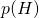, exactly half? And how confident can we be about our answer?

First notice that the question, “Was our coin fair?,” inquires about the underlying system itself, not the data we got. This is a key point …

Key Observation 1: Bayesian analysis attempts to characterize the underlying model or distribution, rather than characterizing the observed data. Of course, the data is used to characterize the model.

If our Bayesian analysis really can tell us about all possiblevalues, then we can assess whether we should believe the value 1/2 or 2/3 or whether we really can’t tell the difference. To start on this process, let’s define the probability of heads by the symbol, then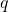represents the underlying distribution, which we’ll call the “model”.

The goal of Bayesian analysis is to estimate the conditional probability of a model (avalue) given the particular data (HHT) that was obtained, denoted by(model | data) … and possibly incorporating prior information about the model. The function(model | data) is called the posterior distribution because it is obtained after the data.

In our case, the posterior distribution we want is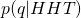, an estimate of relative probabilities for differentvalues given the sequence of flips HHT. If we know the probability of each, not only can we examine the special value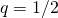but, more importantly, we can see howcompares to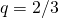or any other value of interest.

Bayesian analysis can be seen a simple application (though confusing to the beginner!) of elementary probability theory, so let’s start with a common sense approach. One thing that we can obviously do is assume a model (avalue) and then calculate the probability of our data (HHT) in that model. To do this we don’t even need to assign a numericalvalue. We can use elementary probability theory: the product of a series of events is simply the product of the individual probabilities. Thus, we have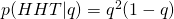, which is true for any. In other words, we have an explicit function for the probability of the data given a model embodied in.The function(HHT|q) is plotted here and looks like what we would expect, with a peak at. That is, there is no othervalue that yields higher probability for the sequence of flips HHT. On the other hand, there are other features that make intuitive sense: the probability to observe HHT givenvalues near zero or one are vanishingly small. All the preceding comments apply explicitly to the probability of seeing the data given the model embodied in.

And yet, all the observations could be “turned around” and qualitatively, they also apply to the posterior distribution we’re seeking. The posterior is the probability ofgiven the data,(q|HHT). Clearly the most likely(in the absence of any other information) must be 2/3. And since we have seen both H and T values in our data, the probability that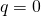or 1 must vanish. So we already seem to be on the right track. At least in our case, we can draw a tentative conclusion …

Key Observation 2: Qualitatively,( model | data )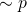( data | model ). That is, it seems we can use easy-to-calculate data probabilities to estimate model probabilities. This point will be formalized below.

We are finally ready to jump into the formal math of the Bayesian approach, which should be a bit easier to appreciate with the HHT example in mind. The Bayesian framework requires us only to understand the following rule of probability for a two-dimensional probability distribution:. Here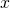andare any variables of interest (continuous or discrete-valued like H and T),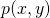is the joint distribution over both variables, meaning thatis the probability in a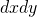square for a continuous distribution andis simply the absolute probability in a discrete system. Sinceis a two-dimensional distribution it must be normalized when integrated (or summed) over allandvalues. Also, we can integrate (sum) over allvalues for eachto get the “marginal” distribution, or vice versa to get the other marginal. See any basic probability reference for more information on these issues.

The Bayesian picture employs the two-dimensional joint distribution of data and models. This is the key conceptual leap, assuming model probabilities can be quantified together in the first place. We can therefore refine our first observation.

Key Observation 1a. The Bayesian picture assumes that there is a true mathematical distribution of models (not just data), and not all models are equally likely.

This is a rather abstract point, and perhaps you may question it. After all, logically, it seems that there must have been only a single model that generated our data – we just don’t know what it was. True, but that single model is assumed to be unknowable (with absolute certainty). Instead, from the statistical perspective, we instead hope only to characterize the likelihood of different underlying models based on the information we have.

Back to coin flipping. To make progress, we simply need to manipulate the rules for two-dimensional probability distributions discussed above in order to get the posterior (distribution for) we want. We write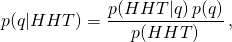which is called Bayes’ rule or formula.

Here, the posterior of interest is on the left. On the right are factors that may be familiar or not. The factoris the simplest – it’s the probability of HHT given a specificvalue, as we already discussed. The factor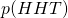technically is the overall probability of HHT in all possible models, but the nice thing about it is that it does not depend on q at all: it’s a constant for anyvalue on the left, so we can think of it as an uninteresting normalization constant.

Most intriguing here is the so-called “prior” distribution ofvalues,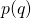. This factor represents our knowledge about whichvalues are likely – independent of the data. For instance, if our HHT data was generated by a real physical coin, perhaps it’s much harder to make a coin exhibiting very small or largevalues (near 0 or 1), so those probabilities might be smaller. Perhaps it’s your big brother using his magic set with unfair coins and you know that he only has coins with the valuesand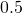.

Let’s look more closely at the example of your big brother, who’s tried to fool you lots of times – and so you know he’s 90% likely to choose the unfair coin () and only 10% likely to choose the fair coin. In that case, given just two coin flips to keep things simple, we can make the following table listing all the joint and marginal probabilities.

 HH HT TH TT Marginal: p(q) q = 0.3 0.3*0.3*0.9 0.3*0.7*0.9 0.7*0.3*0.9 0.7*0.7*0.9 0.9 q = 0.5 0.5*0.5*0.1 0.5*0.5*0.1 0.5*0.5*0.1 0.5*0.5*0.1 0.1 Marginal 0.106 0.214 0.214 0.466

This table is worth staring at for a while. The eight entries in the middle of the table are the estimated posterior probabilities – with proper normalization to make things concrete. The marginalin the right column is the prior.

Try picking a given data value (e.g., HH or TT) and see what the estimatedprobabilities are. This will tell you both the best guess forgiven any particular data, as well as the relative chance you’re wrong, given the assumed prior. If the two flips were TT, for example, then the table shows there is a 95% likelihood (0.441/0.466) that= 0.3. The key thing here is that this is different than the 90% prior: the data have helped point you toward the more likely outcome. (Other pairs of flips make= 0.3 less likely than its prior, as you can check.) More data will help a lot, not surprisingly, as described below.

Let’s build on Bayes’ rule to re-examine the case wherecan take on any value between 0 and 1. The posterior, our estimate for the likelihood of, is given by, where we have omitted the unimportant constant factor. The relation is almost what we hypothesized before from common sense: the probability of the model given the data is proportional to the probability of the data given the model aside from prior information we may have to correct that. But in hindsight, it’s also clear that prior information about possible or likelyvalues must correct our estimate. Thus, we can make another observation.

Key Observation 3: Bayes’ formula tells us, mathematically, how to incorporate “prior” knowledge about the set of (im)possible models. The formula makes intuitive sense, in that the intrinsic, data-independent prior probability of any model acts as a corrective pre-factor or weight for the overall “posterior” estimate of a model’s probability. If we have no prior information about models, then the posterior model probability is simply proportional to the data probability.

Let’s consider whether and when the prior really matters. Perhaps you’ve heard the choice of prior is extremely important – or maybe you’ve heard the prior doesn’t really matter. Well, both are true in different situations.

We need to look more closely at the data probability,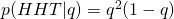. This is just the product of the probabilities for each event, so more generally, we have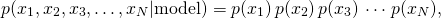where each factor on the right side is really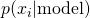.

If each event is a coin toss and there are many of them, then in contrast to thefunction shown above, the full product of probabilities will become a very sharply peaked function of. (You should check this for a sequence containing some arbitrary large numbers of H and T values.) Thus, in the case of large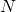, really only a small range ofvalues will be probable. If the peak inis sharp enough, just a tiny range will be probable regardless of the prior. So we can add to our list of observations.

Key Observation 4: The prior distribution (assumption about models) is more important when there is less data and decreasingly important as the amount of data grows.

Maybe your brain is getting tired, even in this briefest of introductions, but there is one more key point about Bayesian statistics.

One of the most important features of the Bayesian formulation is that it inherently brings with it the ability to characterize the range of uncertainty associated with a certain model. In other words, it’s easy to find the most likelyvalue (which was 2/3 for our HHT example), but our original question was whether we think the coin was fair or not.

So what do we think … was the coin fair?!? Of course it’s impossible to answer yes or no based on the data alone, but we can make a simple calculation to quantify the situation.

Let’s take the simple case of a constant prior for now, in which case the posterior distribution ofis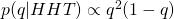. Since we know the distribution for anyvalue, we can simply evaluate it at the two values of interest. We find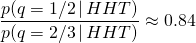That is, with a constant prior, the likelihood that HHT data was generated by a fair coin is 84% as high as the simple guess. There’s quite a good chance the coin was fair.You can take this type of analysis further by determining a range of reasonably probablevalues as sketched above. What range you want to use will depend on what question you are trying to answer. Quantifying a range, however, is a topic beyond the scope of this post. In any case, we can make our final observation.

Key Observation 5: The Bayesian formulation intrinsically quantifies uncertainty in the underlying model. This is because the posterior distribution of models provides an estimate for the likelihood of all models which thus can be compared directly.

Note that standard confidence intervals from frequentist statistics are not direct characterizations of the underlying model in the same way that a Bayesian analysis. Rather, frequentist analysis characterizes ranges of outcomes, as emphasized in an earlier post. To take an extreme case, if you know a coin can be heads or tails but with an unknownvalue – and only a single coin flip is performed yielding H – frequentist statistics really cannot characterize anything about the situation whereas Bayesian analysis can.